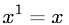Equations > Algebra > Exponents/Powers > Exponent Equal to One Rule

### Exponent Equal to One RuleLatex Code:

MathML Code:

 ${x}^{1}=x$

MathType 5.0: